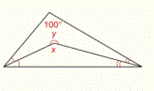Chapter 2.CR, Problem 15CR### Elementary Geometry for College St...

6th Edition
Daniel C. Alexander + 1 other
ISBN: 9781285195698

#### Solutions

Chapter
Section### Elementary Geometry for College St...

6th Edition
Daniel C. Alexander + 1 other
ISBN: 9781285195698
Textbook Problem
577 views

# For Review Exercises 12 to 15 , find the values of x and y .To determine

To find:

The values of x and y.

Explanation

Given:

In ΔABC and ΔABD,

mDBC=mDBAmDAB=mDAC

Figure (1)

Property:

(1) In a triangle the sum of all interior angles is 180°.

(2) Measure of complete angle is 360°.

Calculation:

In ΔABC the sum of all interior angles is 180°.

So,

mC+mDBC+mDAC=180°.

Substitute 100 for mC in the above equation.

100+mDBC+mDAC+mDBA+mDAB=180°mDBC+mDAC+mDBA+mDAB=180°100°mDBC+mDAC+mDBA+mDAB=80°

Substitute mDBC for mDBA and mDAC for mDAB in the above equation.

### Still sussing out bartleby?

Check out a sample textbook solution.

See a sample solution

#### The Solution to Your Study Problems

Bartleby provides explanations to thousands of textbook problems written by our experts, many with advanced degrees!

Get Started

#### Explain what happens during each of the two stages of the two-factor ANOVA.

Essentials of Statistics for The Behavioral Sciences (MindTap Course List)

#### In Exercises 19-24, find the functions f + g, f g, fg, and fg. 24. f(x) = x2 + 1; g(x) = x+1

Applied Calculus for the Managerial, Life, and Social Sciences: A Brief Approach

#### Sometimes, Always, or Never: limxaf(x)g(x)=limxaf(x)limxag(x).

Study Guide for Stewart's Single Variable Calculus: Early Transcendentals, 8th

#### Distinguish between science and pseudoscience.

Research Methods for the Behavioral Sciences (MindTap Course List)